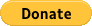﻿﻿﻿﻿﻿ ﻿ Quizzes - discover and download quizzes by SD maths ﻿
.Sitemap  Mobile Version﻿
﻿
﻿﻿﻿

5 quizzesBack to the category list     Sort the quizzes by: nom | date | hitsAsk for the CD-ROM containing all the quizzesDownload Atlence Click'n Study to create your quizzes

Solids by SD maths on 18/11/2011

Be familiar with vocabulary (base, lateral side, top, and so on), be able to associate a pattern to its solid, give the exact name of a pyramid (from its base), and so on.

Angles - Vocabulary by SD maths on 18/11/2011

Fractions - Equality and comparison by SD maths on 18/11/2011

Determine a fraction from simple diagrams. Determine equality of fractions(3 levels: Beginner / Medium / Expert). Test the methods for comparing fractions (2 levels: Beginner / Expert). Simplify fractions (2 levels: Beginner / Expert).

Angles of a triangle by SD maths on 18/11/2011

Calculate angles of different types of triangle (isosceles,...).
Determine if it is a triangle and if so its type from figures or wording.

Basic mathematical formulas by SD maths on 18/11/2011

Summary of the most important formulas for junior high school students.

### Top of the week

1. Past participle agreement of French auxiliary AVOIR
2. Functions and graphs
3. Convert lengths
4. General knowledge
5. Applications of chemistry
6. Friends - Season 1
7. Solids
8. Basic mathematical formulas
9. The Language of Flowers
10. English irregular verbs

### What's new?

1. M.C.Q. general knowledge
2. Computer science exan level 1
3. 10 questions on computer science
4. Capitals of the World Quiz
5. Computer science quiz for junior secondary school
6. English irregular verbs
7. Highway code